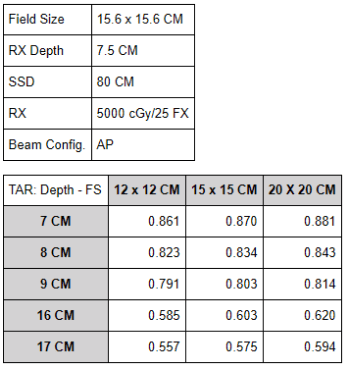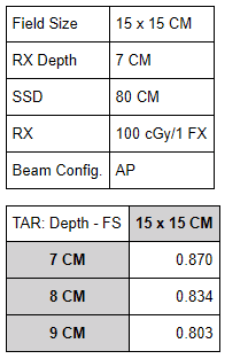The following equations are used for TAR calculations:

1. TAR = dose at depth in tissue (D_dtissx) / dose at depth in air (D_dairx).
2. Equivalent Square = 4 * Area (A) / Perimeter (P).
3. Dose in air at farther point distance = dose in air at closer point distance * (closer point distance /farther point distance) ^2.
4. Dose in air at closer point distance = dose in air at farther point distance * (farther point distance / closer point distance) ^2.
• The last two equations are for the inverse square law calculation in air and the arrangement of the distances (numerator/denominator) will depend on whether you are calculating for a point distance shallower to or deeper to the reference point. Through any medium radiation passes through, the greater depth a beam traverses, the more the beam will depreciate by attenuation. Calculating for a point distance deeper to the reference point will result in a lesser absorbed dose relative to a shallower point. On the contrary, calculating for a point distance shallower to the reference point will result in a greater absorbed dose relative to a deeper point.• Find the total dose to the spinal cord which is at a depth of 16.3 CM
1. Interpolate for TAR at 7 CM depth w/ field size of 15.6 CM: {[(0.881-0.870)/50]*6}+0.870 = 0.871
2. Interpolate for TAR at 8 CM depth w/ field size of 15.6 CM: {[(0.843-0.834)/50]*6}+0.834 = 0.835
3. Interpolate for TAR at 7.5 CM depth w/ field size of 15.6 CM: [(0.835-0.871)/2]+0.871 = 0.853
4. Calculate for dose at depth in air at 7.5 CM depth: 200/D_dair = 0.853; D_dair = 234.47 cGy
5. Calculate for dose at depth in air at 16.3 CM depth using inverse square law: 234.47*(87.5/96.3)^2 = 193.58 cGy
6. Interpolate for TAR at 16 CM depth w/ field size of 15.6 CM: {[(0.620-0.603)/50]*6}+0.603 = 0.605
7. Interpolate for TAR at 17 CM depth w/ field size of 15.6 CM: {[(0.594-0.575)/50]*6}+0.575 = 0.577
8. Interpolate for TAR at 16.3 CM depth w/ field size of 15.6 CM: {[(0.577-0.605)/10]*3}+0.605 = 0.597
9. Find the total dose to the spinal cord at 16.3 CM depth: D_dtiss/193.58 = 0.597; D_dtiss = 115.57*25 fractions = 2889.25 cGy• Find the percent depreciation at depths of 8 CM and 9 CM
1. Calculate for dose at depth in air at 7 CM depth: 100/D_dair = 0.870; D_dair = 114.94 cGy
2. Calculate for dose at depth in air at 8 CM depth using inverse square law: 114.94*(87/88)^2 = 112.34 cGy
3. Find dose to tissue at depth of 8 CM: D_dtiss/112.34 = 0.834; D_dair = 93.69 cGy
4. Find percent depreciation at 8 CM depth: (100-93.69)/100 = 6.31%
5. Find dose to air at depth of 9 CM using inverse square law: 112.34*(88/89)^2 = 109.83 cGy
6. Find dose to tissue at depth of 9 CM: D_dtiss/109.83 = 0.803; D_dtiss = 88.19 cGy

Find percent depreciation at 9 CM depth: (93.69-88.19)/93.69 = 5.87%